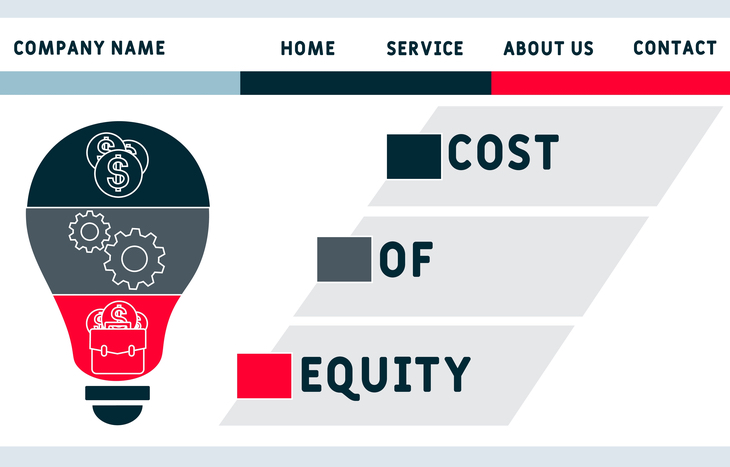How much do you need to make for an investment to be worthwhile? It’s a simple question that every investor needs to consider before they open a position. The answer, no matter what it is, is the cost of equity.

The cost of equity is the rate of return required by the investor to justify the investment. In a stock market filled with public companies to invest in, it’s a simple way to explore how viable an investment is before opening a position. It’s an evaluative formula that investors can use to compare investments, as well as evaluate the efficacy of their investment criteria.

Cost of equity is something companies need to consider, as well. As they seek to distinguish themselves from competitors, companies undertake expansion projects and investments to help them grow. Here too, the cost of equity helps determine the viability of an investment.

Here’s a closer look at this concept and what it means, as well as how it’s applied from both an investor standpoint and from the perspective of a public company.## Breaking Down the Cost of Equity

The cost of equity is the rate of return investors seek to achieve on any given investment. Typically, this comes from one of two sources:

• Share appreciation. As the value of a stock’s share price appreciates over time, investors can exit their position for a profit. If an investor buys 10 shares of ABC Company for \$10, the starting value of their investment is \$100. If the share price climbs to \$20 and they exit the position, they receive \$200 and realize a profit of \$100 total.
• Dividend payments. If the board of directors authorizes a common stock dividend, investors reap the benefits of regular dividend payments. For example, ABC Company may choose to issue a 5% annual dividend. If its shares trade for \$10 and you own 10 shares, you profit \$5 annually, simply for being a shareholder

Share price appreciation and dividend payments both factor into the cost of equity, because they’re the drivers of ROI in that investment. By measuring these two sources of ROI, investors can gauge a company’s ability to meet their expectations for returns.

## Calculating the CoE

Cost of equity is a simple calculation that looks specifically at a stock’s current price, dividend payments and the potential for future dividend growth. The formula is as follows:

CoE = (Dividend / Share Price) + Rate of Appreciation

Investors can use this equation by solving for the cost of equity and matching it up to their desired level of return. For example:

Jose is looking to invest in the telecom sector. He’s looking at AT&T (NYSE: T), which currently trades at \$24 and pays a dividend of 8.5% (\$2.04/share), which has increased at a rate of 4%. Jose wants to see 12% returns on his investment, so he uses the cost of equity formula: (\$2.04 / \$24) + 4% = 12.5%. This investment meets his criteria.

Investors can also use this formula to determine if their expectations are valid. For example, if you didn’t know the growth rate of a company’s dividend, you can plug in your desired cost of equity and solve for the unknown variable. This would tell you the rate at which the dividend needs to grow to meet your requirement. A figure like 4% is much more viable than a figure like 16%, which gives investors some idea of how to set expectations accordingly.

## Cost of Equity vs. The Capital Asset Pricing Model (CAPM)

What happens if the company doesn’t pay a dividend? Investors can instead look at the capital asset pricing model (CAPM) as a more robust way to evaluate the investment worthiness of a company. This formula functions similarly to the cost of equity model to Evaluate the value of an investment based on risk and the time value of money relative to its anticipated return.

CAPM = Risk-Free Rate of Return + Beta × (Market Rate of Return – Risk-Free Rate of Return)

The theory behind CAPM is more complicated than cost of equity, yet it’s broadly applicable to understanding the potential of an investment relative to the same variables.

## Cost of Equity for Investors vs. Companies

Up to this point, we’ve been talking about the cost of equity from an investor standpoint. That is, the rate of return a company needs to provide for it to be a worthwhile investment. This number varies from investor to investor. For instance, someone trying to slightly pace the market with stable investments might look for a cost of equity of 12% against a broad market average of 10%. Meanwhile, someone with an aggressive portfolio in a volatile sector might shoot for a figure closer to 24%.

Looking at the other side, companies need to consider their own cost of equity in order to drive value. This is the rate of return the company needs to realize on a project or investment. For instance, if it borrows money at 6% interest, that’s its cost of equity. The project must return at least that amount (often much more) to justify the expense. Choosing to raise capital via debt vs. equity establishes this figure.

## What Do You Need to Get Out of an Investment?

Before you make an investment, you should always ask yourself what you want to get out of it. It’s not enough to say “profits;” you need to benchmark and understand if the stock you’re investing in has the potential to actually meet that ROI expectation. Calculating the cost of equity will give you a baseline for expectations and help you choose investments that set you up for success.